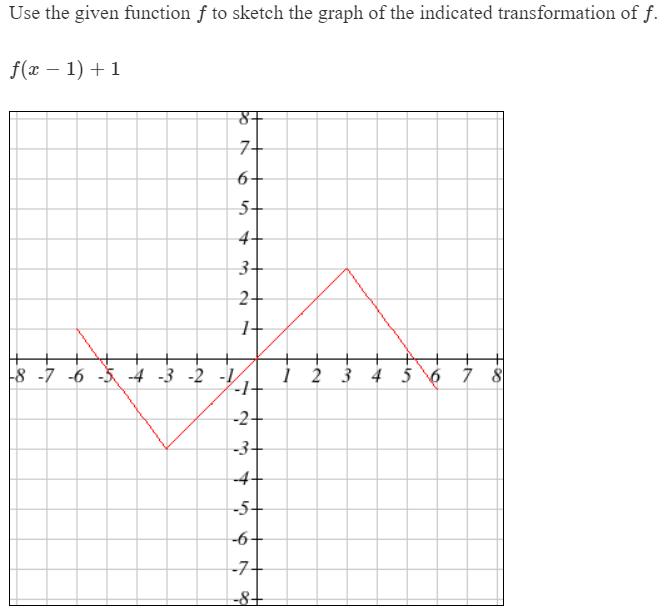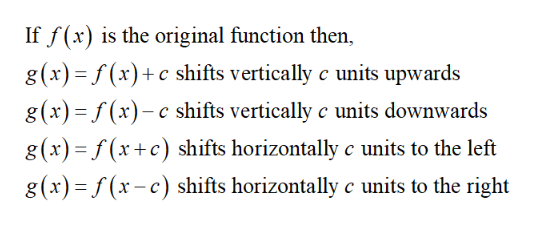# Use the given function f to sketch the graph of the indicated transformation off(x 1)1+8.7+6+5+4+3+2+8 -7 -6 -5 -4 -3 -2 -/2 3 4 5 6 7 8-2+-3+-4-5+-6+-7+-8+

Question
12 viewshelp_outlineImage TranscriptioncloseUse the given function f to sketch the graph of the indicated transformation of f(x 1)1 +8. 7+ 6+ 5+ 4+ 3+ 2+ 8 -7 -6 -5 -4 -3 -2 -/ 2 3 4 5 6 7 8 -2+ -3+ -4 -5+ -6+ -7+ -8+ fullscreen
check_circle

Step 1

Recall the following informati...help_outlineImage TranscriptioncloseIf f(x) is the original function then, g(x) (x)c shifts vertically c units upwards g(x)(x)c shifts vertically c units downwards g(x)f(xc) shifts horizontally c units to the left g(x)(r-c) shifts horizontally c units to the right fullscreen

### Want to see the full answer?

See Solution

#### Want to see this answer and more?

Solutions are written by subject experts who are available 24/7. Questions are typically answered within 1 hour.*

See Solution
*Response times may vary by subject and question.
Tagged in

### Other